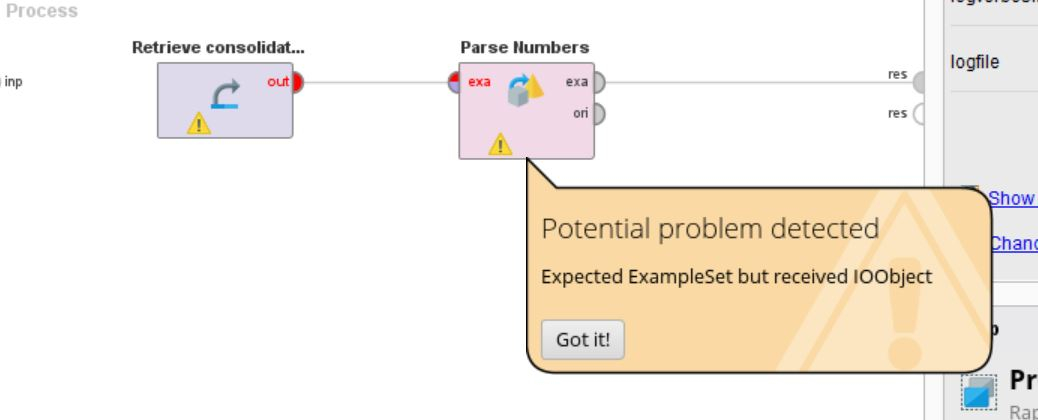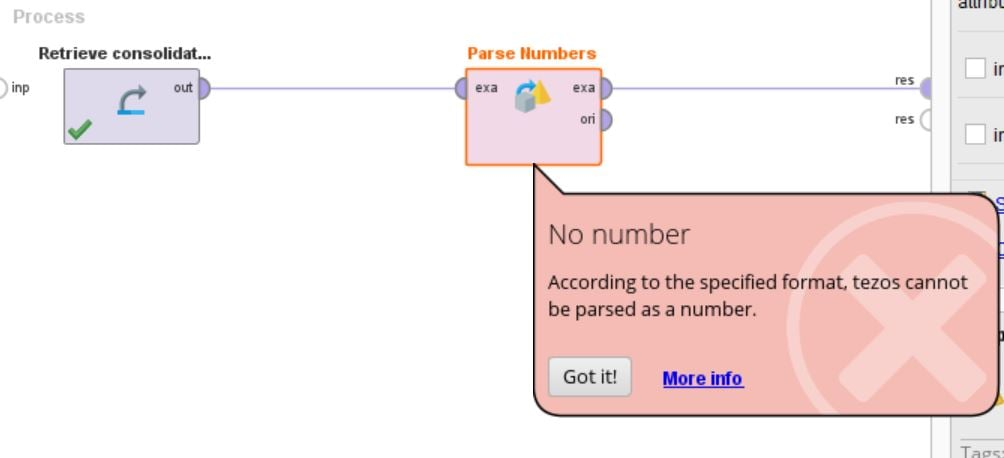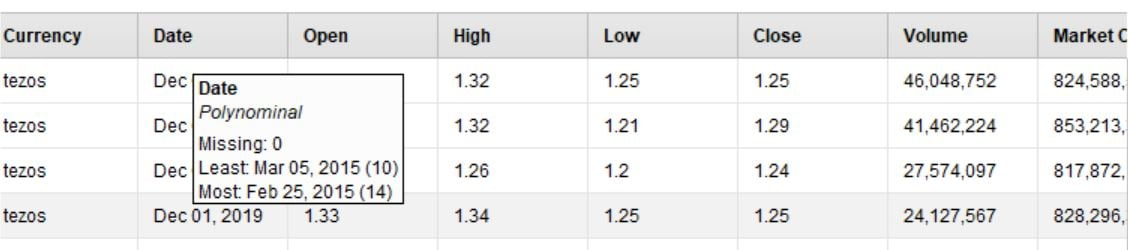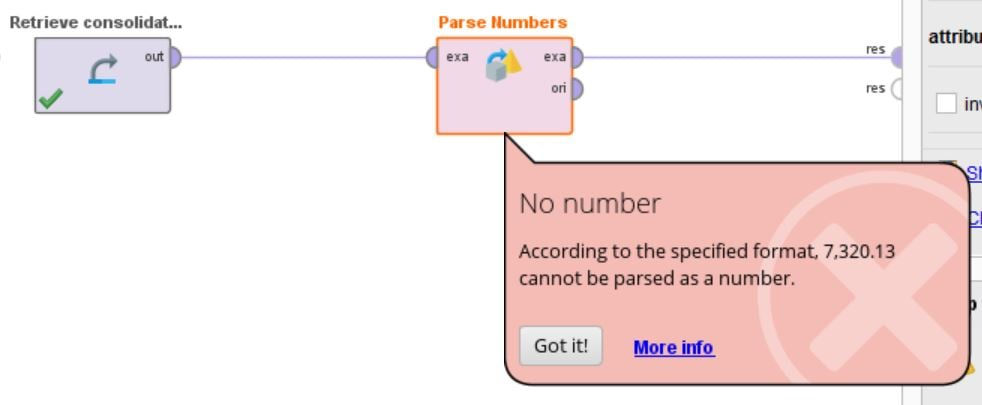# UNPARSEABLE NUMBER ERROR

Member Posts: 9Learner I
Hi,

my data set contained real number data types e.g. 1.290 but it was not accepted when wanting to save and import the dataset. I had to change the real data type to polynomial e.g. 1.29 for it be accepted and imported into rapid miner. Question is why is that?

• Administrator, Employee, RapidMiner Certified Analyst, Member Posts: 271Unicorn
edited July 2020
@SPWM
Try using the Parse Number operator for your attribute. That will try to make the changes and remove any number format that may be affecting the data. Example 1,500.25 will be parsed as 1500.25 and 1.29 will be 1.29.
Maybe there is another data point that is preventing you from saving the attribute as a Real Number
• Member Posts: 9Learner I
that will only work if the data is successfully imported...i am prevented from importing because the real data type, in formatting of columns screen, of 1.290 is not accepted and I have to change it to polynomial data type and only then it will be accepted as imported data into my repository?
• Administrator, Moderator, Employee, RapidMiner Certified Analyst, RapidMiner Certified Expert Posts: 955Unicorn
Hi @SPWM,

you should import the data as nominal, then apply the Parse Numbers operator, and store the result in the repository.

However, there is a "Decimal Character" setting in the import wizard. For importing 1.290 as 1.29 (real) setting that to "." should be sufficient.

Regards,
Balázs
• Member Posts: 9Learner I
Sorry man...I am maybe not communicating properly and new to Rapidminer. When you say I must import the data as nominal, how do i go about this when I cant import the .csv data because the attributes is stored as "real" data type. This is not being accepted if I want to import and will only accept the .csv data into my repository when I change that "real" to "polynomial" data type.
• Administrator, Moderator, Employee, RapidMiner Certified Analyst, RapidMiner Certified Expert Posts: 955Unicorn
Hi,

you're on the right way. Polynominal is a subset of nominal, so you can import as polynominal (not polynomial, that's something entirely different). Then you can use the Parse Numbers operator.

Regards,
Balázs
• Member Posts: 9Learner I
My bad...misspelling the wordThe Parse Numbers operator is used for changing the type of nominal attributes to a numeric type.

if i insert this operator after the imported data is inserted in the new process screen, link the two, I receive the following warning sign:• Member Posts: 9Learner I
Sorry wrong image• Member Posts: 9Learner I
Here is a snippet of the dataok I used subset and excluded currency name and date and I still received warnings• Moderator, RapidMiner Certified Analyst, RapidMiner Certified Expert, Member Posts: 1,635Unicorn
This error is telling you to check the format options of the Parse Number operator.  You have to make sure you set the decimal character and the grouping character properly for your data.
Brian T.
Lindon Ventures
Data Science Consulting from Certified RapidMiner Experts
• Member Posts: 9Learner I
I think I figured it out based on the last solution provided by @Telcontar120
I chose the subset that I had to convert to polynominal (in order for the data to be imported), then checked the box called grouped digits and a comma appeared in the group character...clicked on play and the subset was successfully changed to numeric which I assume is the same as real data type of the original data set imported.

I just cant figure out why it had to be changed to polynominal before being accepted but not as real data type?
Also I cannot understand why the volume and market cap data has comma's e.g 46,048,752, listed as polynominal whereas the subset data are listed with dots e.g 1.290 price data?

Anyone care to maybe set me on the right path and confirm what I did above was correct please?

• Administrator, Employee, RapidMiner Certified Analyst, Member Posts: 271Unicorn
@SPWM the reason you had to import the data as polynomial is due to the way your data was stored on the CSV. The csv file you are uploading saved the numeric data with its formatting and RM takes it as text since formatting is something that is used fior human interpretation.
DB an csv file would store numeric data as only numbers and a decimal separator.
That said number like 46,048,752 or 1.290 are really stored as 46048752 and 1.290
RM helps you "cleaning" the formatting of your numerical attributes with the Parse Number operator and the grouping that could also depende on the locale of each country.
What you are experiencing is part of the Data Cleansing process of Data Minning sometimes you may find a N/A instead of a missing o a 0 or # and by importing data as Polynomial you allow RM to import the data to the software.
it important to define which attributes Parse Number is going to work on when you applied it on the Currency attributed it throwed and exception because there are no numbers on that field.
I hope this helps you understand the logic behind what you had to do.

• Member Posts: 9Learner I
Thank you @MarcoBarradas. Appreciate the explanation.
That said number like 46,048,752 or 1.290 are really stored as 46048752 and 1.290
1.290 remains same?

• Administrator, Employee, RapidMiner Certified Analyst, Member Posts: 271Unicorn
The process creates 3 attributes with 3 decimals, 2 decimals and a Real number. Then I Format them and all the numbers are saved as polynomial in order to keep the grouping.
Then you have 3 ways to convert them back to numbers
First process converts the first attribute to numbers by applying the Parse Numbers operator on that attribute.
Second process tries to Guess the type of the attribute an it does it well for the attributes that only contain decimal point
The third one Parse Numbers on all the attributes indicating there are numbers with formats that need to be parsed.

Hope this little process and example helps you understand whats happening  with the numbers.
```<?xml version="1.0" encoding="UTF-8"?><process version="9.7.001">
<context>
<input/>
<output/>
<macros/>
</context>
<operator activated="true" class="process" compatibility="9.7.001" expanded="true" name="Process">
<parameter key="logverbosity" value="init"/>
<parameter key="random_seed" value="2001"/>
<parameter key="send_mail" value="never"/>
<parameter key="process_duration_for_mail" value="30"/>
<parameter key="encoding" value="SYSTEM"/>
<process expanded="true">
<operator activated="true" class="utility:create_exampleset" compatibility="9.7.001" expanded="true" height="68" name="Create ExampleSet" width="90" x="179" y="34">
<parameter key="generator_type" value="attribute functions"/>
<parameter key="number_of_examples" value="100"/>
<parameter key="use_stepsize" value="false"/>
<list key="function_descriptions">
<parameter key="Decimals3" value="rand()*10"/>
<parameter key="Decimals2" value="round(rand()*10,2)"/>
<parameter key="Real" value="round(rand()*100000000)"/>
</list>
<list key="numeric_series_configuration"/>
<list key="date_series_configuration"/>
<list key="date_series_configuration (interval)"/>
<parameter key="date_format" value="yyyy-MM-dd HH:mm:ss"/>
<parameter key="time_zone" value="SYSTEM"/>
<parameter key="column_separator" value=","/>
<parameter key="parse_all_as_nominal" value="false"/>
<parameter key="decimal_point_character" value="."/>
<parameter key="trim_attribute_names" value="true"/>
</operator>
<operator activated="true" class="format_numbers" compatibility="9.7.001" expanded="true" height="82" name="Format Numbers" width="90" x="313" y="34">
<parameter key="attribute_filter_type" value="all"/>
<parameter key="attribute" value=""/>
<parameter key="attributes" value=""/>
<parameter key="use_except_expression" value="false"/>
<parameter key="value_type" value="numeric"/>
<parameter key="use_value_type_exception" value="false"/>
<parameter key="except_value_type" value="real"/>
<parameter key="block_type" value="value_series"/>
<parameter key="use_block_type_exception" value="false"/>
<parameter key="except_block_type" value="value_series_end"/>
<parameter key="invert_selection" value="false"/>
<parameter key="include_special_attributes" value="false"/>
<parameter key="format_type" value="number"/>
<parameter key="locale" value="English (United States)"/>
<parameter key="use_grouping" value="true"/>
</operator>
<operator activated="true" class="multiply" compatibility="9.7.001" expanded="true" height="124" name="Multiply" width="90" x="447" y="34"/>
<operator activated="true" class="parse_numbers" compatibility="9.7.001" expanded="true" height="82" name="All_to_Numerical" width="90" x="581" y="238">
<parameter key="attribute_filter_type" value="all"/>
<parameter key="attribute" value="Decimals3"/>
<parameter key="attributes" value=""/>
<parameter key="use_except_expression" value="false"/>
<parameter key="value_type" value="nominal"/>
<parameter key="use_value_type_exception" value="false"/>
<parameter key="except_value_type" value="file_path"/>
<parameter key="block_type" value="single_value"/>
<parameter key="use_block_type_exception" value="false"/>
<parameter key="except_block_type" value="single_value"/>
<parameter key="invert_selection" value="false"/>
<parameter key="include_special_attributes" value="false"/>
<parameter key="decimal_character" value="."/>
<parameter key="grouped_digits" value="true"/>
<parameter key="grouping_character" value=","/>
<parameter key="infinity_representation" value=""/>
<parameter key="unparsable_value_handling" value="fail"/>
</operator>
<operator activated="true" class="parse_numbers" compatibility="9.7.001" expanded="true" height="82" name="Parse Numbers" width="90" x="581" y="34">
<parameter key="attribute_filter_type" value="single"/>
<parameter key="attribute" value="Decimals3"/>
<parameter key="attributes" value=""/>
<parameter key="use_except_expression" value="false"/>
<parameter key="value_type" value="nominal"/>
<parameter key="use_value_type_exception" value="false"/>
<parameter key="except_value_type" value="file_path"/>
<parameter key="block_type" value="single_value"/>
<parameter key="use_block_type_exception" value="false"/>
<parameter key="except_block_type" value="single_value"/>
<parameter key="invert_selection" value="false"/>
<parameter key="include_special_attributes" value="false"/>
<parameter key="decimal_character" value="."/>
<parameter key="grouped_digits" value="true"/>
<parameter key="grouping_character" value=","/>
<parameter key="infinity_representation" value=""/>
<parameter key="unparsable_value_handling" value="fail"/>
</operator>
<operator activated="true" class="guess_types" compatibility="9.7.001" expanded="true" height="82" name="Guess Types" width="90" x="581" y="136">
<parameter key="attribute_filter_type" value="all"/>
<parameter key="attribute" value=""/>
<parameter key="attributes" value=""/>
<parameter key="use_except_expression" value="false"/>
<parameter key="value_type" value="attribute_value"/>
<parameter key="use_value_type_exception" value="false"/>
<parameter key="except_value_type" value="time"/>
<parameter key="block_type" value="attribute_block"/>
<parameter key="use_block_type_exception" value="false"/>
<parameter key="except_block_type" value="value_matrix_row_start"/>
<parameter key="invert_selection" value="false"/>
<parameter key="include_special_attributes" value="false"/>
<parameter key="decimal_point_character" value="."/>
</operator>
<connect from_op="Create ExampleSet" from_port="output" to_op="Format Numbers" to_port="example set input"/>
<connect from_op="Format Numbers" from_port="example set output" to_op="Multiply" to_port="input"/>
<connect from_op="Multiply" from_port="output 1" to_op="Parse Numbers" to_port="example set input"/>
<connect from_op="Multiply" from_port="output 2" to_op="Guess Types" to_port="example set input"/>
<connect from_op="Multiply" from_port="output 3" to_op="All_to_Numerical" to_port="example set input"/>
<connect from_op="All_to_Numerical" from_port="example set output" to_port="result 3"/>
<connect from_op="Parse Numbers" from_port="example set output" to_port="result 1"/>
<connect from_op="Guess Types" from_port="example set output" to_port="result 2"/>
<portSpacing port="source_input 1" spacing="0"/>
<portSpacing port="sink_result 1" spacing="0"/>
<portSpacing port="sink_result 2" spacing="0"/>
<portSpacing port="sink_result 3" spacing="0"/>
<portSpacing port="sink_result 4" spacing="0"/>
</process>
</operator>
</process>
```

• Member Posts: 9Learner I
@MarcoBarradas thank you for the explanation, appreciate it. I am not a coder and learning as I go along. Would you recommend me to learn Python or R or an easy method to transition into code using Rapidminer? What about Integer Operator, would that not serve the same purpose as Parse Operator?